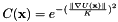# Anisotropic Diffusion Perona Malik

Hi there,

I have two questions related to the anisotropic diffusion filters (gradient and curvature) in the SimpleITK library. Specifically,

1. Do the filters do any scaling to the conductance parameter, K, that you provide to the filters? i.e. if I provide a K value of 2, does 2 get fed directly into the equation or does it get scaled with the range of pixel intensities, for example?

2. Is the K value used constant or do the filters recalculate K every iteration as described by Perona and Malik (using 90% of the cumulative distribution function of gradient values) in the referenced paper below and in the filter documentation (also linked to below)? i.e. if I give a value of 2 with 10 iterations, is 2 used every iteration or do the filters automatically adjust K as the image changes with each iteration?

I looked through the source code and couldn’t figure either of these out definitively.

The reference is listed here: https://itk.org/Doxygen/html/classitk_1_1AnisotropicDiffusionFunction.html
The reference is: Pietro Perona and Jitendra Malik, `‘Scale-space and edge detection using anisotropic diffusion,’’ IEEE Transactions on Pattern Analysis Machine Intelligence, vol. 12, pp. 629-639, 1990

Thanks in advance for any insight!

1 Like

Hello @michael,

The SimpleITK library is primarily a simplified wrapper of ITK. Were you looking at the ITK source code? That is where the actual work happens.

It looks like the k value is recalculated every iteration

Admittedly it is not easy to manually trace things in the ITK code (inheritance and overriding…), but
that is the nature of a big library, and likely easier to do within an IDE.

2 Likes

Hi @zivy,

Thanks for your response! Yes, I was looking at the ITK code on GitHub as you linked to in your response. As you mentioned, it is pretty difficult (at least for me) to follow the source code and really understand what the filter is doing. This is my first time working with ITK and image processing in general so I apologize if anything I say is particularly naive.

To be clear in the rest of this post, when I say “K”, I am referring to the K in this equation from the documentationand “conductance term” refers to C(x) in the same equation. “Conductance parameter” is only used as it appears in the code, for example in the line of code shown below we see GetConductanceParameter() which grabs the value m_ConductanceParameter.

So, if I am understanding everything correctly, I input a value of K (as m_ConductanceParameter based on the code here). m_ConductanceParameter is then squared and multiplied by -2*AverageGradientMagnitudeSquared in the line of code you linked to (and shown below) to get m_K:

``````       InitializeIteration() override  {
m_K = static_cast<PixelType>(this->GetAverageGradientMagnitudeSquared() * this->GetConductanceParameter() *
this->GetConductanceParameter() * -2.0f);  }
``````

m_K is then used to calculate the conductance term, C(x), shown here:

``````Cx = std::exp((itk::Math::sqr(dx_forward) + accum) / m_K);
``````

C(x) is then used to calculate the smoothing based on the diffusion equation. On the next iteration, the InitializeIteration() function uses the first value of m_K (calculated above) with “this->GetConductanceParameter()”, which is then used to calculate the new value of m_K for the next iteration.

So, given all of this, here are my concrete questions:

1. Is everything I described above correct?

2. Is it correct to say that filter is not using the 90% method described by Perona and Malik to recalculate K every iteration? Instead, it is rescaling K using the equation in InitializeIteration().

3. Where does the -2 come from in InitializeIteration()? It seems like that equation has all the ingredients frombut I can’t reconcile it.

4. One thing I notice is that if I calculate the initial input value of K using the method by Perona and Malik, I will get different results from the filter if I rescale the input image. But I will get the same result if I use the same K value. So for example, if my original image has an pixel intensity range of 0-32000, I may get a K value by Perona and Malik of around 3000. If I rescale the same image to 0-255, I get a K value around 25 - so proportionally the same relative K value. If I run both of these images through the filter with the same number of iterations and timestep, and their respective K values, I get different end results. However, if I do the same thing with the same K value (say 3 which is the default value of the filter) for both images, the end results are the same. My interpretation of this is that since the filter is automatically adjusting the K value (via InitializeIteration()) to the scaling of the image, I need to adjust the calculated K value (via Perona-Malik or other literature methods which are dependent on the absolute scaling of the image) into what I will call the “standard K scaling” used by the filter. For example, if I divide the K value obtained from Perona and Malik by the average gradient magnitude, I get a value within the range listed in the documentation (0.5-2.0), but that range is given with reference to the Visible Human color data, so I’m not sure it is relevant to my images necessarily. Does this interpretation sound correct? Do you have any insight into this “standard K scaling” of the filter?

I apologize for the lengthy response, but I wanted to make sure everything was clear. Thanks again in advance for any insight!